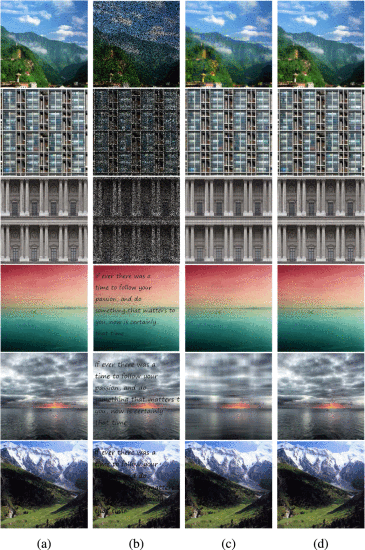# Nonconvex Nonsmooth Low-Rank Minimization via Iteratively Reweighted Nuclear Norm### Abstract

The nuclear norm is widely used as a convex surrogate of the rank function in compressive sensing for low rank matrix recovery with its applications in image recovery and signal processing. However, solving the nuclear norm-based relaxed convex problem usually leads to a suboptimal solution of the original rank minimization problem. In this paper, we propose to use a family of nonconvex surrogates of L 0 -norm on the singular values of a matrix to approximate the rank function. This leads to a nonconvex nonsmooth minimization problem. Then, we propose to solve the problem by an iteratively re-weighted nuclear norm (IRNN) algorithm. IRNN iteratively solves a weighted singular value thresholding problem, which has a closed form solution due to the special properties of the nonconvex surrogate functions. We also extend IRNN to solve the nonconvex problem with two or more blocks of variables. In theory, we prove that the IRNN decreases the objective function value monotonically, and any limit point is a stationary point. Extensive experiments on both synthesized data and real images demonstrate that IRNN enhances the low rank matrix recovery compared with the state-of-the-art convex algorithms.

Type
Publication
IEEE Transactions on Image Processing